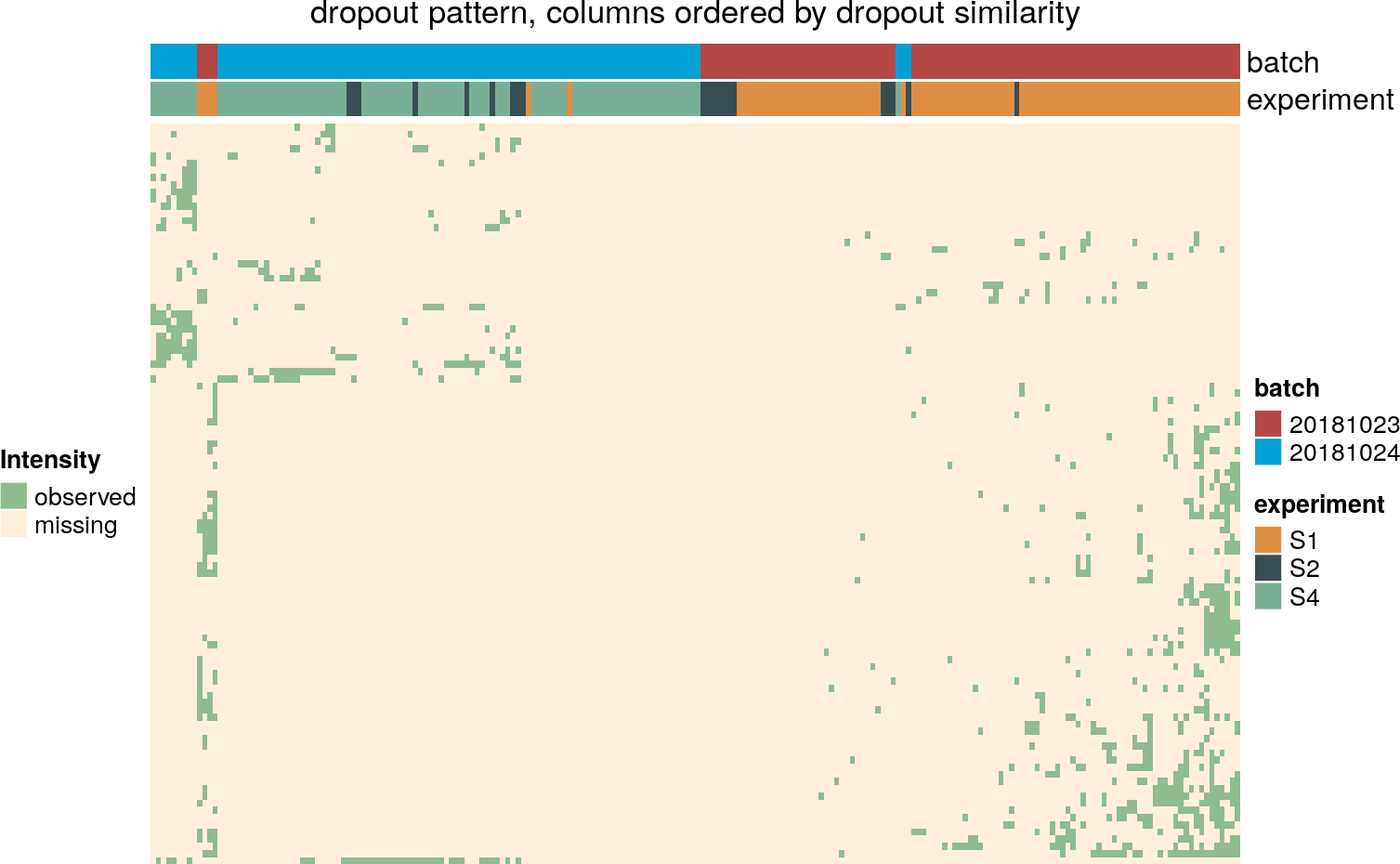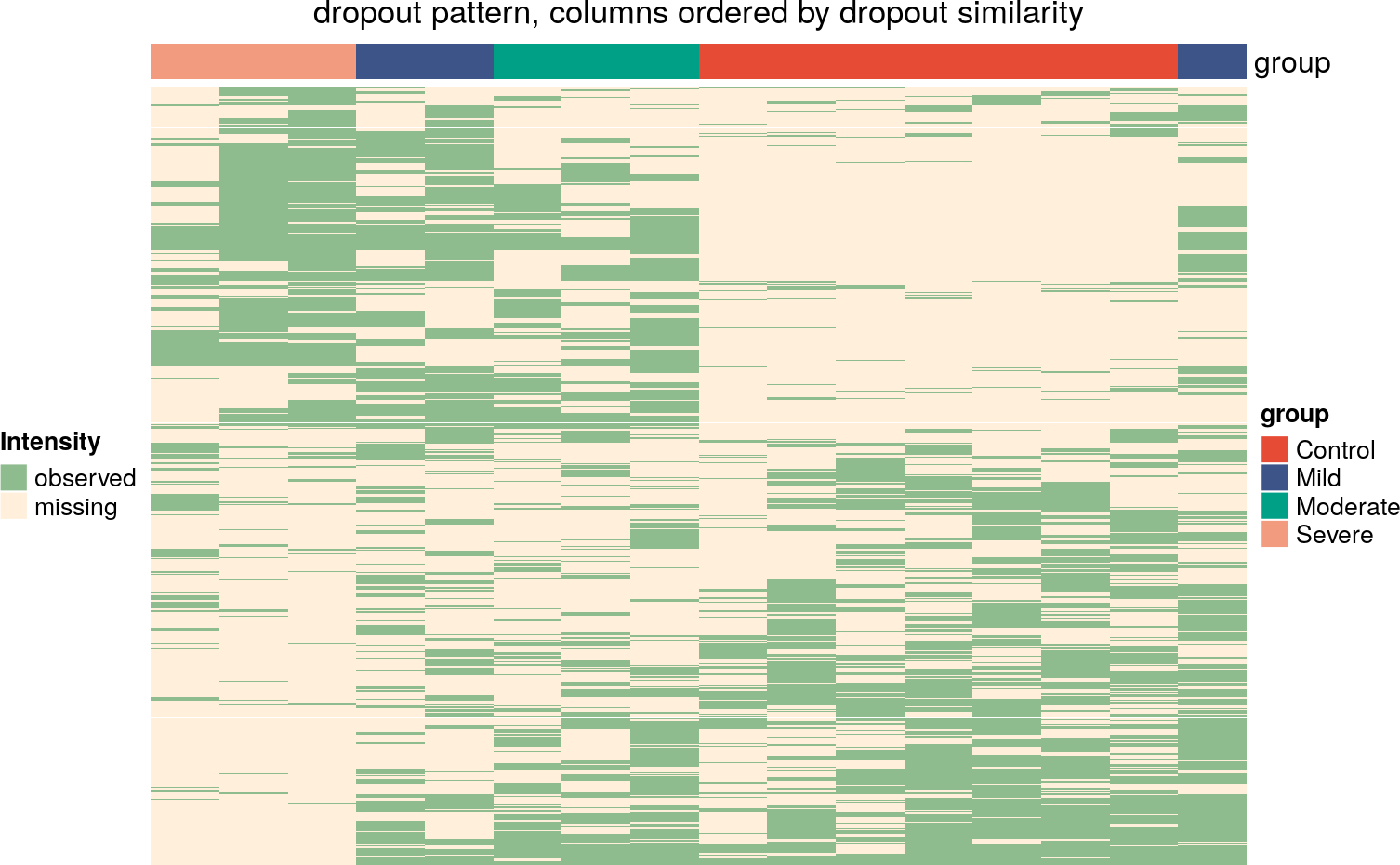1 Installation

if(!requireNamespace("BiocManager", quietly = TRUE))
install.packages("BiocManager")
BiocManager::install("msImpute")

2 Quick Start

The package consists of the following main functions:

• selectFeatures: identifies informative peptides that can be used to examine MAR/MNAR missingness in the data.

• msImpute: Main function that imputes missing values by learning a low-rank approximation of the data.

• findVariableFeatures: finds peptide with high biological variance. We use this in computeStructuralMetrics

• computeStructuralMetrics: returns a number of metrics that measure distortions into the data after imputation.

• plotCV2: Plots the square of coefficient of variation versus average log-expression i.e. mean-\(CV^2\) plot

These functions overall are designed to inform user’s decision in choosing a proper imputation strategy. For a more detailed workflow, please see User’s Manual.

3 TIMS Case Study: Blood plasma

The aim is to assess the missing patterns in ion mobility data by Prianichnikov et al. (2020), available from PXD014777. The evidence table of MaxQuant output was processed as described below. Rows are Modified Peptide IDs. Charge state variations are treated as distinct peptide species. For peptides with multiple identification types, the intensity is considered to be the median of reported intensity values. Reverse complements and contaminant peptides are discarded. Peptides with more than 4 observed intensity values are retained.

The data was acquired in two batches (over two days). We are interested to know if missing values are evenly distributed across batches, or there is a batch-specific dropout trend. The runs are also labeled by S1, S2 and S4 (source unknown). The aim is to use this information to work out if missing values occur due to technical or biological effects.

library(reticulate)
library(msImpute)
library(limma)
library(imputeLCMD)
library(ComplexHeatmap)

3.1 Data processing

The following procedures were applied to process the data, which we later load from the package data.

3.1.1 Filter by detection

data(pxd014777)
y <- pxd014777

Zero values that will be converted to Inf/-Inf after log- transformation. Check if there are valid values in the data before log transformation

table(is.infinite(data.matrix(log2(y))))
##
##  FALSE   TRUE
## 610515    681

There are zero values that will be converted to Inf/-Inf after log- transformation. Add a small offset to avoid infinite values:

y <- log2(y+0.25)

3.1.2 Normalization

# quantile normalisation
y <- normalizeBetweenArrays(y, method = "quantile")

3.2 Determine missing values pattern

Determine dominant patterns of missing values by investigating the distribution of missing values. Peptides that are missing in at least one experimental group (here batch), and therefore exhibit structured missing patterns can be identified by the EBM metric implemented in selectFeatures. We then make a heatmap of their dropout pattern.

batch <- as.factor(gsub("(2018.*)_RF.*","\\1", colnames(y)))
experiment <- as.factor(gsub(".*(S[1-9]).*","\\1", colnames(y)))

hdp <- selectFeatures(y, method = "ebm", group = batch)

# peptides missing in one or more experimental group will have a NaN EBM, which is a measure of entropy of
# distribution of observed values
table(is.nan(hdp\$EBM))
##
## FALSE  TRUE
##  2780   103
# construct matrix M to capture missing entries
M <- ifelse(is.na(y),1,0)
M <- M[hdp\$msImpute_feature,]

# plot a heatmap of missingness patterns for the selected peptides
ha_column <- HeatmapAnnotation(batch = batch,
experiment = experiment,
col = list(batch = c('20181023' = "#B24745FF",
'20181024'= "#00A1D5FF"),
experiment=c("S1"="#DF8F44FF",
"S2"="#374E55FF",
"S4"="#79AF97FF")))

hm <- Heatmap(M,
column_title = "dropout pattern, columns ordered by dropout similarity",
name = "Intensity",
col = c("#8FBC8F", "#FFEFDB"),
show_row_names = FALSE,
show_column_names = FALSE,
cluster_rows = TRUE,
cluster_columns = TRUE,
show_column_dend = FALSE,
show_row_dend = FALSE,
top_annotation = ha_column,
row_names_gp =  gpar(fontsize = 7),
column_names_gp = gpar(fontsize = 8),
heatmap_legend_param = list(#direction = "horizontal",
heatmap_legend_side = "bottom",
labels = c("observed","missing"),
legend_width = unit(6, "cm")),
)
hm <- draw(hm, heatmap_legend_side = "left")Figure 1: Heatmap of missing value patterns for peptides selected as informative peptides

The larger the EBM, the more scattered the missing values will be. If missing values are scattered across samples, their value can be estimated from the neighborhood, hence missing type is likely MNAR. If however, peptides are missing completely in one experimental condition, or they have much more concentrated (or dense) distributions, their EBM value will be lower. A NaN EBM suggests peptide is missing in at least one experimental group, defined by the group argument. Since there are 103 such peptides with EBM=NaN, this data has peptides that are missing not at random i.e. the missingness is batch-specific. Given that this is a technical dataset, MNAR missing here can not be biological, and reflects batch-to-batch variations, such as differences in limit of detection of MS etc. selectFeatures just enables to detect any peptides that appear to exhibit structured missing, and hence might be left-censored. you can also set method="hvp" which will select top n_features peptides with high dropout rate, defined as proportion of samples where a given peptide is missing, that are also highly expressed as the msImpute_feature in the output dataframe. If method="ebm", the features marked in msImpute_feature column will be peptides (or proteins, depending on the input expression matrix), will the ones with NaN EBM (i.e. peptides with structured missing patterns). The "hvp" method can detect missingness patterns at high abundance, whereas "ebm" is for detection of peptides (completely) missing in at least one experimental group.

4 DDA Case Study: Extracellular vesicles isolated from inflammatory bowel disease patients and controls

The study aims to characterize the proteomic profile of extracellular vesicles isolated from the descending colon of pediatric patients with inflammatory bowel disease and control participants. The following analysis is based on the peptide table from MaxQuant output, available from PXD007959. Rows are Modified Peptide IDs. Charge state variations are treated as distinct peptide species. Reverse complements and contaminant peptides are discarded. Peptides with more than 4 observed intensity values are retained. Additionally, qualified peptides are required to map uniquely to proteins. Two of the samples with missing group annotation were excluded.

4.1 Filter by detection

The sample descriptions can be accessed via pxd007959\$samples. Intensity values are stored in pxd007959\$y.

data(pxd007959)

sample_annot <- pxd007959\$samples
y <- pxd007959\$y
y <- log2(y)

4.2 Normalization

We apply cyclic loess normalisation from limma to normalise log-intensities. We have justified use of cyclic loess method in depth in the user’s guide.

y <- normalizeBetweenArrays(y, method = "cyclicloess")

4.3 Determine missing values pattern

# determine missing values pattern
group <- sample_annot\$group
hdp <- selectFeatures(y, method="ebm", group = group)
# construct matrix M to capture missing entries
M <- ifelse(is.na(y),1,0)
M <- M[hdp\$msImpute_feature,]

# plot a heatmap of missingness patterns for the selected peptides
ha_column <- HeatmapAnnotation(group = as.factor(sample_annot\$group),
col=list(group=c('Control' = "#E64B35FF",
'Mild' = "#3C5488FF",
'Moderate' = "#00A087FF",
'Severe'="#F39B7FFF")))

hm <- Heatmap(M,
column_title = "dropout pattern, columns ordered by dropout similarity",
name = "Intensity",
col = c("#8FBC8F", "#FFEFDB"),
show_row_names = FALSE,
show_column_names = FALSE,
cluster_rows = TRUE,
cluster_columns = TRUE,
show_column_dend = FALSE,
show_row_dend = FALSE,
top_annotation = ha_column,
row_names_gp =  gpar(fontsize = 7),
column_names_gp = gpar(fontsize = 8),
heatmap_legend_param = list(#direction = "horizontal",
heatmap_legend_side = "bottom",
labels = c("observed","missing"),
legend_width = unit(6, "cm")),
)
hm <- draw(hm, heatmap_legend_side = "left")Figure 2: Dropout pattern of informative peptides

As it can be seen, samples from the control group cluster together. There is a structured, block-wise pattern of missing values in the ‘Control’ and ‘Severe’ groups. This suggests that missing in not at random. This is an example of MNAR dataset. Given this knowledge, we impute using QRILC and msImpute, setting method to v2-mnar. We then compare these methods by preservation of local (within experimental group) and global (between experimental group) similarities. Note that low-rank approximation generally works for data of MAR types. However, the algorithm implemented in v2-mnar makes it applicable to MNAR data. To make low-rank models applicable to MNAR data, we need to use it in a supervised mode, hence why we need to provide information about groups or biological/experimental condition of each sample.

4.4 Imputation

# imputation

y_qrilc <- impute.QRILC(y)[]

y_msImpute <- msImpute(y, method = "v2-mnar", group = group)
## Running msImpute version v2-mnar
## rank is 12
## computing lambda0 ...
## lambda0 is 545.047266965271
## fit the low-rank model ...
## model fitted.
## Imputting missing entries ...
## Imputation completed
group <- as.factor(sample_annot\$group)

4.5 Assessment of preservation of local and global structures

If you’ve installed python, and have set up a python environment in your session, you can run this section to compute the GW distance. Please see the user’s guide for setup instructions. Note that you can still run computeStructuralMetrics by setting y=NULL, if there are no python environments setup.

Withinness, betweenness and Gromov-Wasserstein (GW) distance

computeStructuralMerics returns three metrics that can be used to compare various imputation procedures:

• withinness is the sum of the squared distances between samples from the same experimental group (e.g. control, treatment, Het, WT). More specifically the similarity of the samples is measured by the distance of the (expression profile of the) sample from group centroid. This is a measure of preservation of local structures.

• betweenness is the sum of the squared distances between the experimental groups, more specifically the distance between group centroids. This is a measure of preservation of global structures.

• gw_dist is the Gromov-Wasserstein distance computed between Principal Components of imputed and source data. It is a measure of how well the structures are overall preserved over all principal axis of variation in the data. Hence, it captures preservation of both local and global structures. PCs of the source data are computed using highly variable peptides (i.e. peptides with high biological variance).

An ideal imputation method results in smaller withinness, larger withinness and smaller gw_dist among other imputation methods.

virtualenv_create('msImpute-reticulate')
virtualenv_install("msImpute-reticulate","scipy")
virtualenv_install("msImpute-reticulate","cython")
virtualenv_install("msImpute-reticulate","POT")

use_virtualenv("msImpute-reticulate")

top.hvp <- findVariableFeatures(y)

computeStructuralMetrics(y_msImpute, group, y[rownames(top.hvp)[1:50],], k = 16)
Computing GW distance using k= 16 Principal Components
\$withinness
Mild  Control Moderate   Severe
10.39139 11.53781 10.54993 10.46477

\$betweenness
 11.50008

\$gw_dist
 0.01717915
computeStructuralMetrics(y_qrilc, group, y[rownames(top.hvp)[1:50],], k = 16)
Computing GW distance using k= 16 Principal Components
\$withinness
Mild  Control Moderate   Severe
10.34686 11.84049 10.62378 10.73958

\$betweenness
 11.62664

\$gw_dist
 0.008877501

Withinness tends to be smaller by msImpute, which indicates that local structures are better preserved by these two methods. The gw_dist over all PCs for the two methods is very similar (rounded to 2 decimals). This suggests the enhancements in v2-mnar is just as good as left-censored MNAR methods such as QRILC. Note that k is set to the number of samples to capture all dimensions of the data.

Also note that that, unlike QRILC, msImpute v2-mnar dose not drastically increase the variance of peptides (measured by squared coefficient of variation) post imputation.

par(mfrow=c(2,2))
pcv <- plotCV2(y, main = "data")
pcv <- plotCV2(y_msImpute, main = "msImpute v2-mnar")
pcv <- plotCV2(y_qrilc, main = "qrilc")